# Need to convert Date into a particular formatHi,

I have a line item formatted as date in the module like: "4/29/2020" and i want it to convert into "As of Apr 29, 2020". I tried myself with the below formula but didn't work, kindly advise:

"As of " & TEXT(MONTH(As of Date)) & "/" & TEXT(DAY(As of Date)) & "/" & TEXT(YEAR(As of Date))

Thank you!

Tagged:

•Sorry I applied this formula:
"As of " & TEXT(MONTH(As of Date)) & " " & TEXT(DAY(As of Date)) & "," & TEXT(YEAR(As of Date))
•but i have period in weeks
•that's why in my solution I use an intermediary LI so you can format it as the time period you want (month here)
•I got the solution but this is not the way I am looking for, if possible please suggest me an appropriate way of doing this, below is the approach I followed:

I created another line item where i applied the below formula to get the month name and then concatenated with other values:
"IF MONTH(Date) = 1 THEN "January" ELSE IF MONTH(Date) = 2 THEN "February" ELSE IF MONTH(Date) = 3 THEN "March" ELSE IF MONTH(Date) = 4 THEN "April" ELSE IF MONTH(Date) = 5 THEN "May" ELSE IF MONTH(Date) = 6 THEN "June" ELSE IF MONTH(Date) = 7 THEN "July" ELSE IF MONTH(Date) = 8 THEN "August" ELSE IF MONTH(Date) = 9 THEN "September" ELSE IF MONTH(Date) = 10 THEN "October" ELSE IF MONTH(Date) = 11 THEN "November" ELSE "December""
•This is not necessary - will be taxing to the model performance. Just break the formula as I said earlier you will be able to see the output

You can change the Time period from months to weeks in intermediate line item and then use that  in final line item

•@Misbah is correct in having that as long IF/THEN/ELSE statement is not good.  I know I am late to the party, but think about doing the following:

Create a Month List with the code be the number of the month: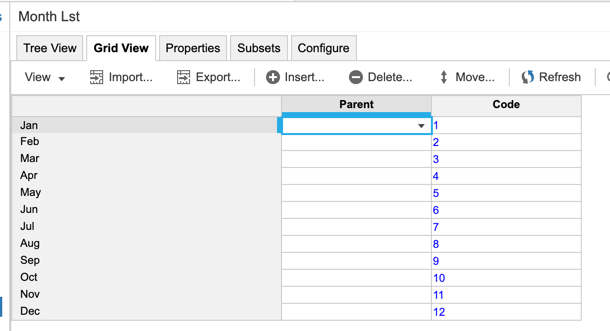Create a SYS Month List Properties module with the following: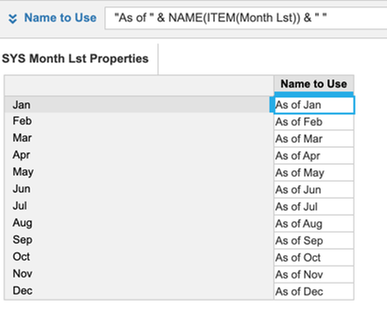*Note - I am adding the "As of " and space after the month here because now the concatenation is only being done 12 times.  Not only is this better, but it will lead to less "&" in the final result.

In a SYS Time - Year module (only dimensionalized by Time at the Year Level), have the following line items: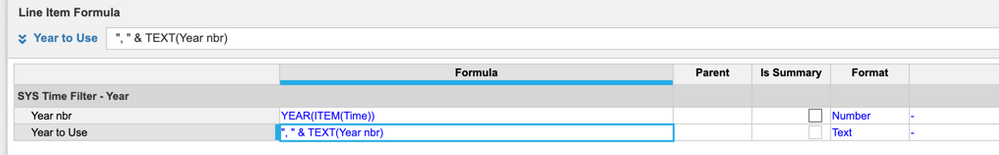*Note - I am adding the comma and space here because in my model, I only have 2 years so the concatenation is only being done twice.

In a SYS Time - Day module (only dimensionalized by Time at the day Level), have the following line items: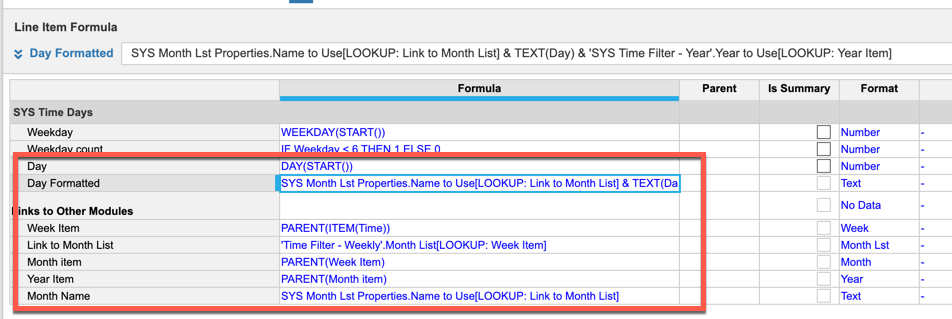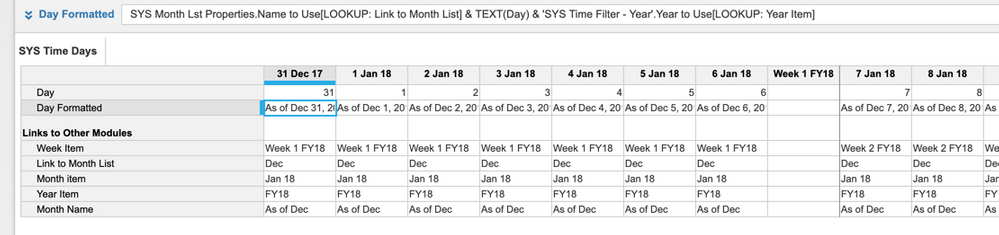So, you might be asking why am I breaking this up?  There are a couple of reasons:

• Performance will be much better here because I only have 3 concatenations whereas you had 5 because I have added the spaces, commas, etc to the line item at the SYS level vs in a module that has many other lists.  Concatenations are performance killers.
• In doing my concatenations on SYS modules, it means they are being done less times and now you can reference the already calculated results from any module that has time or a date.
• This now opens it for other model builders to get to the same data whereas if you had your long IF THEN ELSE statement tucked away in a module, not only would performance be poor, but other model builders would not know where to find this logic.

Hope this helps,

Rob